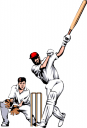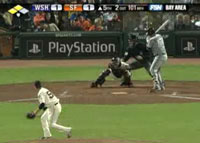## Posts Tagged ‘cricket vs baseball’## Which sport is more DANGEROUS to play? Cricket or baseball?

April 22, 2019Reposted due to popular demand.

Mathspigs you are about to be hit on the head with some BALL PARK figures. There is so much maths involved in comparing cricket and baseball I hardly know where to begin. So I will just set you up with comparative statistics for starters. Here they are:You now have sufficient information to calculate the following:Q1. If a cricket player  makes 4 runs (and runs between the wickets) and a baseball player hits a home run, who runs the furtherest?

ANS: 4 runs in cricket distance = 80.48 m

Home run in baseball distance = 109.6 m

Q2. What are the delivery ball speeds of an average pitcher and a mid paced bowler in m/sec?

ANS: Average Bowler Ball Speed = 110 – 125 km/h = 30.5 – 34.7 m/sec

Average pitcher Ball Speed = 80.47 km/h = 22.4 m/sec

Q3. How far would the delivery of an average pitcher and a  medium paced bowler travel in 10 seconds?

S = d/t

t = 10 secs:

d = S x t = S x 10

ANS: In 10 sec a ball bowled by an average bowler would travel 305 – 347 M

In 10 sec a ball thrown by an average pitcher would travel 224 mQ4. If Shoaib Akhar fielded a ball in cricket 20 m from the wicket when the batsman was still 8m from the wicket (A typical running speed for humans is 32 km/h) would he be able to run him out?

Akhar ball speed S = 45.0 m/sec

d = 20 m

S = d/t

t = d/S

t  = 20/ 45.0

t = 0.44 secs

Batsman Speed = 32 km/h = 8.9 m/sec

How far can the batsman run in 0.44 secs?

d = S x t

d = 8.9 x 0.44 = 3.9 m

So batsman would be easily run out by Akhar as he is 8 m from the crease.

Q5. How long would it take a ball thrown by Joel Zumaya to reach the batsman if the pitchers mound was exactly in the middle of the baseball square?

ANS: Speed of ball pitched by Zumaya = 168.65 km/h = 46.8 m/secQ6. Which sport is more dangerous?

We will base our calculations on the speed (or velocity) and the weight (or mass) of the ball. We must use the same units in all calculations. For each sport plot x-axis: velocity of ball (v) against y-axis mass x velocity (mv) of the ball. mv is the momentum. In ballistics the change in momentum is called the IMPULSE (impact for us). If you are hit on the head with a ball it is the change in momentum of the ball that does the damage. A small ball at a high speed can do as much damage as a larger ball at a slower speed. We are not including spin, curve balls or air resistance to keep calculations straight forward … literally!!!Q6a. Which sport for professional players is the most dangerous?

You will have to look at the graph. A cricket ball is heavier than a baseball BUT the fastest pitchers can pitch a ball slightly faster than bowlers can bowl.  IMPACT (mv) depends on multiplying mass by velocity. You will see the stats for Akhar and Zumaya marked on the graph and you can use these stats to estimate impact.

BUT then there is the impact of batting, which increases the velocity of the ball. That will have to wait for another post.

Q6b. At what speed does a cricket ball do the same damage as a baseball? Give one example.  (Hint: Draw a straight line from the Y-axis)Once again look at the  graph above!

You will find more maths on impact on Mathspig:  for a punch here and from hailstones here.

Pics: Tim Lincecum (Left) with 101 mph pitch, Shane Warne (Upper Right) and Shoaib Akhtar (Lower Left)## Which sport is more DANGEROUS to play? Cricket or baseball?

September 4, 2009Mathspigs you are about to be hit on the head with some BALL PARK figures. There is so much maths involved in comparing cricket and baseball I hardly know where to begin. So I will just set you up with comparative statistics for starters. Here they are:You now have sufficient information to calculate the following:Q1. If a cricket player  makes 4 runs (and runs between the wickets) and a baseball player hits a home run, who runs the furtherest?

ANS: 4 runs in cricket distance = 80.48 m

Home run in baseball distance = 109.6 m

Q2. What are the delivery ball speeds of an average pitcher and a mid paced bowler in m/sec?

ANS: Average Bowler Ball Speed = 110 – 125 km/h = 30.5 – 34.7 m/sec

Average pitcher Ball Speed = 80.47 km/h = 22.4 m/sec

Q3. How far would the delivery of an average pitcher and a  medium paced bowler travel in 10 seconds?

S = d/t

t = 10 secs:

d = S x t = S x 10

ANS: In 10 sec a ball bowled by an average bowler would travel 305 – 347 M

In 10 sec a ball thrown by an average pitcher would travel 224 mQ4. If Shoaib Akhar fielded a ball in cricket 20 m from the wicket when the batsman was still 8m from the wicket (A typical running speed for humans is 32 km/h) would he be able to run him out?

Akhar ball speed S = 45.0 m/sec

d = 20 m

S = d/t

t = d/S

t  = 20/ 45.0

t = 0.44 secs

Batsman Speed = 32 km/h = 8.9 m/sec

How far can the batsman run in 0.44 secs?

d = S x t

d = 8.9 x 0.44 = 3.9 m

So batsman would be easily run out by Akhar as he is 8 m from the crease.

Q5. How long would it take a ball thrown by Joel Zumaya to reach the batsman if the pitchers mound was exactly in the middle of the baseball square?

ANS: Speed of ball pitched by Zumaya = 168.65 km/h = 46.8 m/secQ6. Which sport is more dangerous?

We will base our calculations on the speed (or velocity) and the weight (or mass) of the ball. We must use the same units in all calculations. For each sport plot x-axis: velocity of ball (v) against y-axis mass x velocity (mv) of the ball. mv is the momentum. In ballistics the change in momentum is called the IMPULSE (impact for us). If you are hit on the head with a ball it is the change in momentum of the ball that does the damage. A small ball at a high speed can do as much damage as a larger ball at a slower speed. We are not including spin, curve balls or air resistance to keep calculations straight forward … literally!!!Q6a. Which sport for professional players is the most dangerous?

You will have to look at the graph. A cricket ball is heavier than a baseball BUT the fastest pitchers can pitch a ball slightly faster than bowlers can bowl.  IMPACT (mv) depends on multiplying mass by velocity. You will see the stats for Akhar and Zumaya marked on the graph and you can use these stats to estimate impact.

BUT then there is the impact of batting, which increases the velocity of the ball. That will have to wait for another post.

Q6b. At what speed does a cricket ball do the same damage as a baseball? Give one example.  (Hint: Draw a straight line from the Y-axis)Once again look at the  graph above!

You will find more maths on impact on Mathspig:  for a punch here and from hailstones here.

Pics: Tim Lincecum (Left) with 101 mph pitch, Shane Warne (Upper Right) and Shoaib Akhtar (Lower Left)